Next: Time Differential SR (TD-SR) Up: SR Spectroscopy Previous: SR Spectroscopy   Contents

# Introduction

Muons (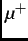,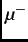) can be produced from the parity-violating decay of pions (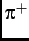,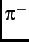), which are produced from the collision of intermediate energy range (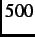-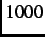MeV) protons  with a low atomic number target consisting of carbon or beryllium nuclei. Positive pions stopping near the downstream surface of the production target spontaneously decay after a mean life time of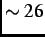ns via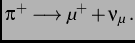(3.1)

Muons created in this way are called surface muons''. Since the neutrino is a left-handed particle (i.e. with its spin is directed antiparallel to its momentum direction) with spin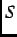=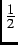and the pion at rest has a spin= 0, conservation of linear and angular momentum requires that the decay muon has its spin pointing in a direction opposite to its linear momentum in the pion rest frame. By collecting the muons traveling in the same direction, a beam with relatively low kinetic energy (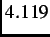MeV) and a nearly 100% spin polarization is obtained. Thus, unlike conventional nuclear magnetic resonance (NMR),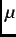SR benefits from a large initial spin polarization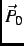, independent of the sample or sample environment. Furthermore, as a=probe, the muon has no electric quadrupole moment and hence is not directly sensitive to electric field gradients.

The muon has a mean lifetime of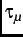=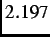s , after which it decays as follows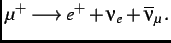(3.2)

The probability of a decay positron with energy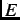emerging with a momentum at an angle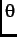with respect to the initial muon spin direction is(3.3a)

where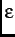=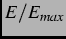is the reduced energy,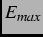=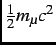=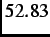MeV is the maximum possible relativistic positron energy and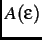is the asymmetry factor. As shown in Fig. 2.1, the distribution of decay positrons is asymmetric. The asymmetry is a maximum (i.e.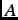=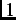) when=, in which case no positrons are emitted antiparallel to the muon spin direction. The high energy positrons easily pass through components of the experimental apparatus (e.g. cryostat walls). They are detected by counters consisting of plastic scintillators, light guides and photomultipliers. The detection of the asymmetric distribution of decay positrons provides a means of determining the time evolution of the spin polarization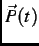associated with an implanted ensemble of muons.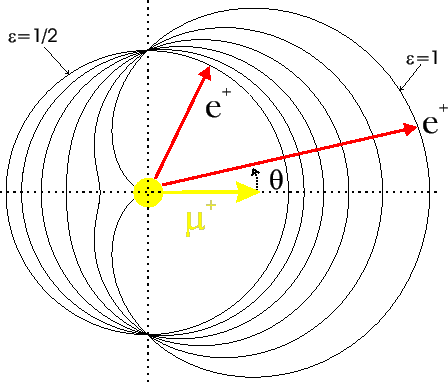Next: Time Differential SR (TD-SR) Up: SR Spectroscopy Previous: SR Spectroscopy   Contents
Jess H. Brewer 2003-07-01